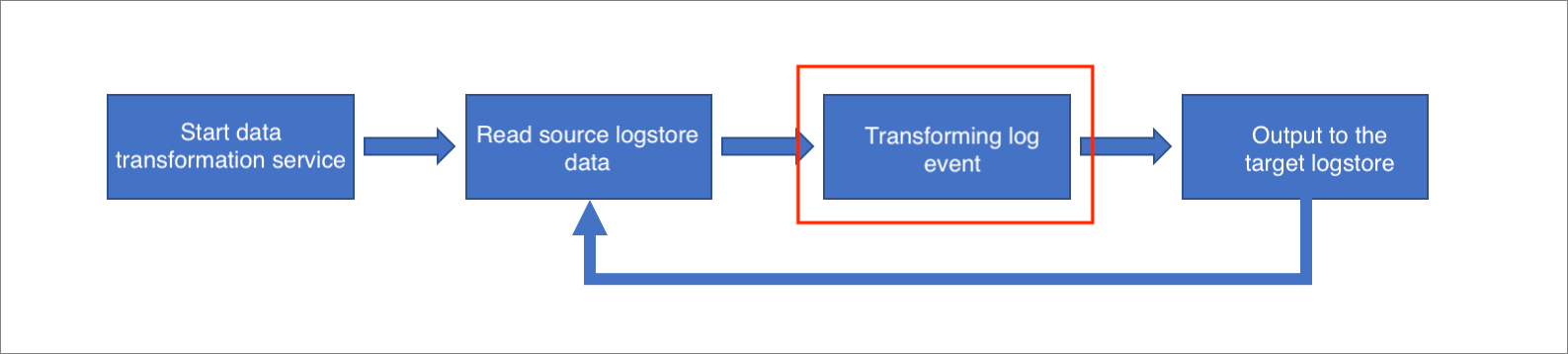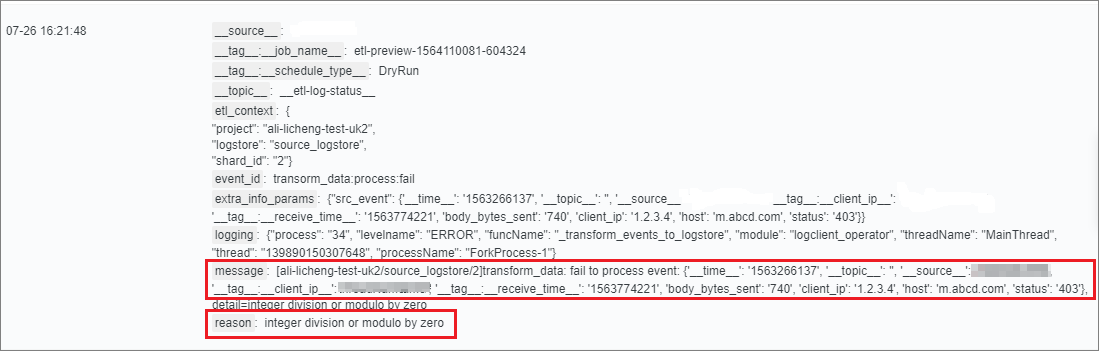This topic describes the causes for errors in data transformation rules and the troubleshooting methods.

After reading data from the source Logstore, the data transformation engine starts to transform log events of the source Logstore.• During this process, the transformation rules may not apply to all or some log events, causing logic errors.
• If the transformation rules involve loading data from external resources such as ApsaraDB for Relational Database Service (RDS) instances and Logstores, errors may also occur in loading or updating data.

## Error impact

• During log event transformation, log events that conflict with the transformation rules cause errors and are discarded. Therefore, the transformation result does not include these log events.
• The transformation task discards log events that conflict with the transformation rules without re-transforming them and continues to transform other log events.
• If multiple events are split from one event, when one of the events is discarded due to an error, all other events that are split from the same source event as this event are also discarded.
Note Before the result is returned, the events are associated with each other based on a tree structure and are not independent of each other.

## Troubleshooting methods

• Check the `message` field of the error log to find the log events with errors. For more information, see View error logs.
• Check the `reason` field of the error log to determine the causes of errors for these log events.
Based on the causes, add logic to transform the log events with errors. You can use the process control functions such as `e_if` and `e_switch` to capture and process the errors.## Common errors and troubleshooting methods

• Log events contain abnormal values.
Sample transformation rule 1:
``````# The value of field b in some log events is 0, causing the error of division by 0.
e_set("c", op_div_floor(v("a"), v("b")))``````
• Error log:
``````{
"reason": "error when calling : floordiv\nDetail: integer division or modulo by zero",
}``````
• Troubleshooting method:

Check whether the value of field `b` in the log events with errors is 0. If so, the error of division by 0 occurs.

• Solution:
The error occurs only when the value of field `b` in a log event is 0. In this case, you can use the `e_if` function to capture field `b` whose value is 0.
``e_if_else(op_eq(v("b"), "0"), e_set("c", v("a")), e_set("c", op_div_floor(v("a"), v("b")))``
Sample transformation rule 2:
``````# The value of field a in some log events is an invalid timestamp, causing an error.
e_set("b", dt_fromtimestamp(v("a")))``````
• Error log:
``````{
"reason": "error when calling : int\nDetail: invalid literal for int() with base 10: 'abnormal value'",
}``````
• Troubleshooting method:

Check whether the value of field `a` in the log events with errors is a valid timestamp (numeric string).

• Solution:
Add logic to check whether the value of field `a` in a log event is a valid timestamp. If not, export the log event to the target Logstore `target2`.
``e_if_else(str_isdigit(v("a")), e_set("b", dt_fromtimestamp(v("a"))), e_output("target2"))``
• The data type is not converted before the numeric operation.
Sample transformation rule:
``````e_set("a", 10)
e_set("b", 10)
e_set("c", op_mul(v("a"), v("b")))``````
• Error log:
``````{
"reason": "error when calling : mul\nDetail: can't mulltiply sequence by non-int of type' str'",
}``````
• Error cause:

In the processing logic of LOG domain specific language (DSL), the values of all fields in log events are stored as strings. In the preceding sample transformation rule, the values of `v("a")` and `v("b")` are strings. If they are directly passed to the `op_mul` function, an error is returned.

• Troubleshooting method:

Check the LOG DSL rules to determine whether the data type is converted before the numeric operation.

• Solution:
Use the `ct_int` function to convert the values from the string type to the integer type before passing them to the `op_mul` function.
``````e_set("a", 10)
e_set("b", 10)
e_set("c", op_mul(c_int(v("a")), c_int(v("b"))))``````